## Get Started with Cascade Object Detector

### Why Train a Detector?

The `vision.CascadeObjectDetector` System object comes with several pretrained classifiers for detecting frontal faces, profile faces, noses, eyes, and the upper body. However, these classifiers are not always sufficient for a particular application. Computer Vision Toolbox™ provides the `trainCascadeObjectDetector` function to train a custom classifier.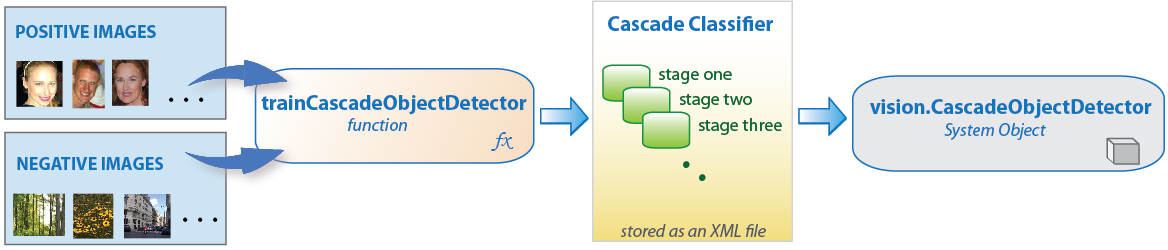### What Kinds of Objects Can You Detect?

The Computer Vision Toolbox cascade object detector can detect object categories whose aspect ratio does not vary significantly. Objects whose aspect ratio remains fixed include faces, stop signs, and cars viewed from one side.

The `vision.CascadeObjectDetector` System object detects objects in images by sliding a window over the image. The detector then uses a cascade classifier to decide whether the window contains the object of interest. The size of the window varies to detect objects at different scales, but its aspect ratio remains fixed. The detector is very sensitive to out-of-plane rotation, because the aspect ratio changes for most 3-D objects. Thus, you need to train a detector for each orientation of the object. Training a single detector to handle all orientations will not work.

### How Does the Cascade Classifier Work?

The cascade classifier consists of stages, where each stage is an ensemble of weak learners. The weak learners are simple classifiers called decision stumps. Each stage is trained using a technique called boosting. Boosting provides the ability to train a highly accurate classifier by taking a weighted average of the decisions made by the weak learners.

Each stage of the classifier labels the region defined by the current location of the sliding window as either positive or negative. Positive indicates that an object was found and negative indicates no objects were found. If the label is negative, the classification of this region is complete, and the detector slides the window to the next location. If the label is positive, the classifier passes the region to the next stage. The detector reports an object found at the current window location when the final stage classifies the region as positive.

The stages are designed to reject negative samples as fast as possible. The assumption is that the vast majority of windows do not contain the object of interest. Conversely, true positives are rare and worth taking the time to verify.

• A true positive occurs when a positive sample is correctly classified.

• A false positive occurs when a negative sample is mistakenly classified as positive.

• A false negative occurs when a positive sample is mistakenly classified as negative.

To work well, each stage in the cascade must have a low false negative rate. If a stage incorrectly labels an object as negative, the classification stops, and you cannot correct the mistake. However, each stage can have a high false positive rate. Even if the detector incorrectly labels a nonobject as positive, you can correct the mistake in subsequent stages.

The overall false positive rate of the cascade classifier is ${f}^{s}$, where $f$ is the false positive rate per stage in the range (0 1), and $s$ is the number of stages. Similarly, the overall true positive rate is ${t}^{s}$, where $t$ is the true positive rate per stage in the range (0 1]. Thus, adding more stages reduces the overall false positive rate, but it also reduces the overall true positive rate.

### Create a Cascade Classifier Using the `trainCascadeObjectDetector`

Cascade classifier training requires a set of positive samples and a set of negative images. You must provide a set of positive images with regions of interest specified to be used as positive samples. You can use the Image Labeler to label objects of interest with bounding boxes. The Image Labeler outputs a table to use for positive samples. You also must provide a set of negative images from which the function generates negative samples automatically. To achieve acceptable detector accuracy, set the number of stages, feature type, and other function parameters.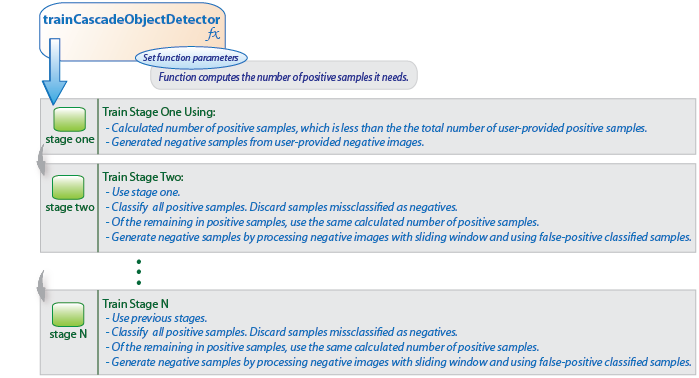#### Considerations when Setting Parameters

Select the function parameters to optimize the number of stages, the false positive rate, the true positive rate, and the type of features to use for training. When you set the parameters, consider these tradeoffs.

ConditionConsideration
A large training set (in the thousands).Increase the number of stages and set a higher false positive rate for each stage.
A small training set.Decrease the number of stages and set a lower false positive rate for each stage.
To reduce the probability of missing an object.Increase the true positive rate. However, a high true positive rate can prevent you from achieving the desired false positive rate per stage, making the detector more likely to produce false detections.
To reduce the number of false detections.Increase the number of stages or decrease the false alarm rate per stage.

#### Feature Types Available for Training

Choose the feature that suits the type of object detection you need. The `trainCascadeObjectDetector` supports three types of features: Haar, local binary patterns (LBP), and histograms of oriented gradients (HOG). Haar and LBP features are often used to detect faces because they work well for representing fine-scale textures. The HOG features are often used to detect objects such as people and cars. They are useful for capturing the overall shape of an object. For example, in the following visualization of the HOG features, you can see the outline of the bicycle.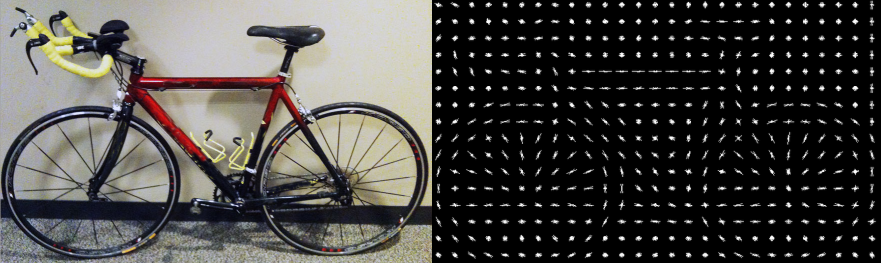You might need to run the `trainCascadeObjectDetector` function multiple times to tune the parameters. To save time, you can use LBP or HOG features on a small subset of your data. Training a detector using Haar features takes much longer. After that, you can run the Haar features to see if the accuracy improves.

#### Supply Positive Samples

To create positive samples easily, you can use the Image Labeler app. The Image Labeler provides an easy way to label positive samples by interactively specifying rectangular regions of interest (ROIs).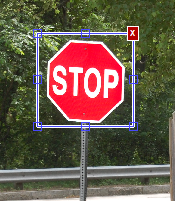You can also specify positive samples manually in one of two ways. One way is to specify rectangular regions in a larger image. The regions contain the objects of interest. The other approach is to crop out the object of interest from the image and save it as a separate image. Then, you can specify the region to be the entire image. You can also generate more positive samples from existing ones by adding rotation or noise, or by varying brightness or contrast.

#### Supply Negative Images

Negative samples are not specified explicitly. Instead, the `trainCascadeObjectDetector` function automatically generates negative samples from user-supplied negative images that do not contain objects of interest. Before training each new stage, the function runs the detector consisting of the stages already trained on the negative images. Any objects detected from these image are false positives, which are used as negative samples. In this way, each new stage of the cascade is trained to correct mistakes made by previous stages.As more stages are added, the detector's overall false positive rate decreases, causing generation of negative samples to be more difficult. For this reason, it is helpful to supply as many negative images as possible. To improve training accuracy, supply negative images that contain backgrounds typically associated with the objects of interest. Also, include negative images that contain nonobjects similar in appearance to the objects of interest. For example, if you are training a stop-sign detector, include negative images that contain road signs and shapes similar to a stop sign.

#### Choose the Number of Stages

There is a trade-off between fewer stages with a lower false positive rate per stage or more stages with a higher false positive rate per stage. Stages with a lower false positive rate are more complex because they contain a greater number of weak learners. Stages with a higher false positive rate contain fewer weak learners. Generally, it is better to have a greater number of simple stages because at each stage the overall false positive rate decreases exponentially. For example, if the false positive rate at each stage is 50%, then the overall false positive rate of a cascade classifier with two stages is 25%. With three stages, it becomes 12.5%, and so on. However, the greater the number of stages, the greater the amount of training data the classifier requires. Also, increasing the number of stages increases the false negative rate. This increase results in a greater chance of rejecting a positive sample by mistake. Set the false positive rate (`FalseAlarmRate`) and the number of stages, (`NumCascadeStages`) to yield an acceptable overall false positive rate. Then you can tune these two parameters experimentally.

Training can sometimes terminate early. For example, suppose that training stops after seven stages, even though you set the number of stages parameter to 20. It is possible that the function cannot generate enough negative samples. If you run the function again and set the number of stages to seven, you do not get the same result. The results between stages differ because the number of positive and negative samples to use for each stage is recalculated for the new number of stages.

#### Training Time of Detector

Training a good detector requires thousands of training samples. Large amounts of training data can take hours or even days to process. During training, the function displays the time it took to train each stage in the MATLAB® Command Window. Training time depends on the type of feature you specify. Using Haar features takes much longer than using LBP or HOG features.

### Troubleshooting

#### What if you run out of positive samples?

The `trainCascadeObjectDetector` function automatically determines the number of positive samples to use to train each stage. The number is based on the total number of positive samples supplied by the user and the values of the `TruePositiveRate` and `NumCascadeStages` parameters.

The number of available positive samples used to train each stage depends on the true positive rate. The rate specifies what percentage of positive samples the function can classify as negative. If a sample is classified as a negative by any stage, it never reaches subsequent stages. For example, suppose you set the `TruePositiveRate` to `0.9`, and all of the available samples are used to train the first stage. In this case, 10% of the positive samples are rejected as negatives, and only 90% of the total positive samples are available for training the second stage. If training continues, then each stage is trained with fewer and fewer samples. Each subsequent stage must solve an increasingly more difficult classification problem with fewer positive samples. With each stage getting fewer samples, the later stages are likely to overfit the data.

Ideally, use the same number of samples to train each stage. To do so, the number of positive samples used to train each stage must be less than the total number of available positive samples. The only exception is that when the value of `TruePositiveRate` times the total number of positive samples is less than 1, no positive samples are rejected as negatives.

The function calculates the number of positive samples to use at each stage using the following formula:

number of positive samples = `floor`(`totalPositiveSamples` / (1 + (`NumCascadeStages` - 1) * (1 - `TruePositiveRate`)))

This calculation does not guarantee that the same number of positive samples are available for each stage. The reason is that it is impossible to predict with certainty how many positive samples will be rejected as negatives. The training continues as long as the number of positive samples available to train a stage is greater than 10% of the number of samples the function determined automatically using the preceding formula. If there are not enough positive samples the training stops and the function issues a warning. The function also outputs a classifier consisting of the stages that it had trained up to that point. If the training stops, you can add more positive samples. Alternatively, you can increase `TruePositiveRate`. Reducing the number of stages can also work, but such reduction can also result in a higher overall false alarm rate.

#### What to do if you run out of negative samples?

The function calculates the number of negative samples used at each stage. This calculation is done by multiplying the number of positive samples used at each stage by the value of `NegativeSamplesFactor`.

Just as with positive samples, there is no guarantee that the calculated number of negative samples are always available for a particular stage. The `trainCascadeObjectDetector` function generates negative samples from the negative images. However, with each new stage, the overall false alarm rate of the cascade classifier decreases, making it less likely to find the negative samples.

The training continues as long as the number of negative samples available to train a stage is greater than 10% of the calculated number of negative samples. If there are not enough negative samples, the training stops and the function issues a warning. It outputs a classifier consisting of the stages that it had trained up to that point. When the training stops, the best approach is to add more negative images. Alternatively, you can reduce the number of stages or increase the false positive rate.

### Train Stop Sign Detector

Load the positive samples data from a MAT file. The file contains the ground truth, specified as table of bounding boxes for several object categories. The grount truth was labeled and exported from the `Image Labeler` app.

`load('stopSignsAndCars.mat');`

Prefix the fullpath to the stop sign images.

`stopSigns = fullfile(toolboxdir('vision'),'visiondata',stopSignsAndCars{:,1});`

Create datastores to load the ground truth data for stop signs.

```imds = imageDatastore(stopSigns); blds = boxLabelDatastore(stopSignsAndCars(:,2));```

Combine the image and box label datastores.

`positiveInstances = combine(imds,blds);`

Add the image folder path to the MATLAB path.

```imDir = fullfile(matlabroot,'toolbox','vision','visiondata','stopSignImages'); addpath(imDir);```

Specify a folder for negative images.

`negativeFolder = fullfile(matlabroot,'toolbox','vision','visiondata','nonStopSigns');`

Create an imageDatastore object containing negative images.

`negativeImages = imageDatastore(negativeFolder);`

Train a cascade object detector called `'stopSignDetector.xml'` using HOG features. NOTE: The command can take several minutes to run.

`trainCascadeObjectDetector('stopSignDetector.xml',positiveInstances,negativeFolder,FalseAlarmRate=0.1,NumCascadeStages=5);`
```Automatically setting ObjectTrainingSize to [35, 32] Using at most 42 of 42 positive samples per stage Using at most 84 negative samples per stage --cascadeParams-- Training stage 1 of 5 [........................................................................] Used 42 positive and 84 negative samples Time to train stage 1: 0 seconds Training stage 2 of 5 [........................................................................] Used 42 positive and 84 negative samples Time to train stage 2: 0 seconds Training stage 3 of 5 [........................................................................] Used 42 positive and 84 negative samples Time to train stage 3: 2 seconds Training stage 4 of 5 [........................................................................] Used 42 positive and 84 negative samples Time to train stage 4: 6 seconds Training stage 5 of 5 [........................................................................] Used 42 positive and 17 negative samples Time to train stage 5: 9 seconds Training complete ```

Use the newly trained classifier to detect a stop sign in an image.

`detector = vision.CascadeObjectDetector('stopSignDetector.xml');`

`img = imread('stopSignTest.jpg');`

`bbox = step(detector,img);`

Insert bounding box rectangles and return the marked image.

` detectedImg = insertObjectAnnotation(img,'rectangle',bbox,'stop sign');`

Display the detected stop sign.

`figure;`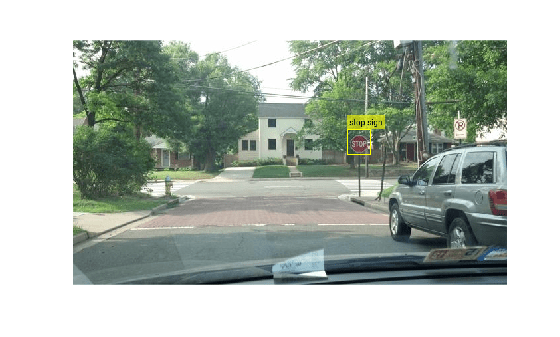`imshow(detectedImg);`

Remove the image folder from the path.

`rmpath(imDir);`